# PSEB 6th Class Maths Solutions Chapter 3 Playing with Numbers Ex 3.3

Punjab State Board PSEB 6th Class Maths Book Solutions Chapter 3 Playing with Numbers Ex 3.3 Textbook Exercise Questions and Answers.

## PSEB Solutions for Class 6 Maths Chapter 3 Playing with Numbers Ex 3.3

1. Find prime factors of the following numbers by factor tree method:

Question (i)
96
Solution: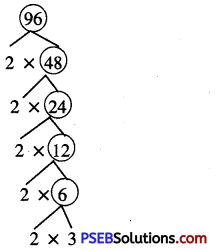∴ Prime factorisation of 96 = 2 × 2 × 2 × 2 × 2 × 3Question (ii)
120
Solution: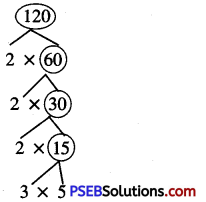∴ Prime factorisation of 120 = 2 × 2 × 2 × 3 × 5

Question (iii)
180.
Solution: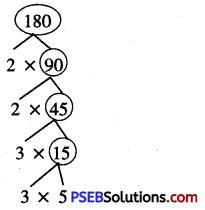∴ Prime factorisation of 180 = 2 × 2 × 3 × 3 × 5

2. Complete each factor tree:

Question (i)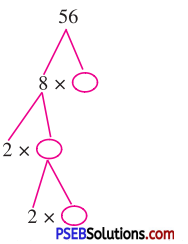Solution: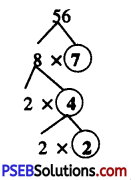Question (ii)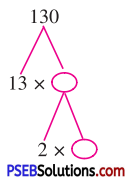Solution: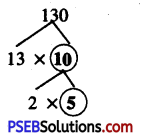Question (iii)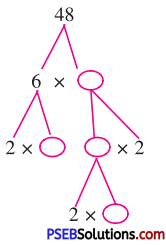Solution: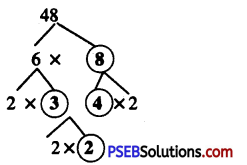3. Find the prime factors of the following numbers by division method:

Question (i)
420
Solution:∴ 420 = 2 × 2 × 3 × 5 × 7

Question (ii)
980
Solution: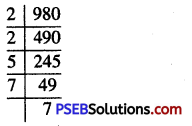∴ 980 = 2 × 2 × 5 × 7 × 7

Question (iii)
225
Solution: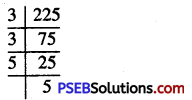∴ 225 = 3 × 3 × 5 × 5Question (iv)
150
Solution: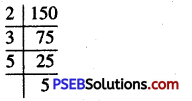∴ 150 = 2 × 3 × 5 × 5

Question (v)
324
Solution: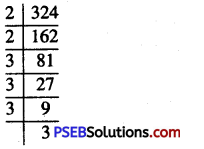∴ 324 = 2 × 2 × 3 × 3 × 3 × 3